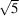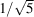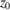International
Tables for
Crystallography
Volume A
Space-group symmetry
Edited by M. I. Aroyo

International Tables for Crystallography (2015). Vol. A, ch. 1.4, pp. 67-68

## Section 1.4.5.1. Introduction

B. Souvignierd

#### 1.4.5.1. Introduction

| top | pdf |

A two-dimensional section of a crystal pattern takes out a slice of a crystal pattern. In the mathematical idealization, this slice is regarded as a two-dimensional plane, allowing one, however, to distinguish its upper and lower side. Depending on how the slice is oriented with respect to the crystal lattice, the slice will be invariant by translations of the crystal pattern along zero, one or two linearly independent directions. A section resulting in a slice with two-dimensional translational symmetry is called a rational section and is by far the most important case for crystallography.

Because the slice is regarded as a two-sided plane, the sym­metries of the full crystal pattern that leave the slice invariant fall into two types:

 (i) If a symmetry operation of the slice maps its upper side to the upper side, a vector normal to the slice is fixed. (ii) If a symmetry operation of the slice maps the upper side to the lower side, a vector normal to the slice is mapped to its opposite and the slice is turned upside down.

Therefore, the symmetries of two-dimensional rational sections are described by layer groups, i.e. subgroups of space groups with a two-dimensional translation lattice. Layer groups are sub­periodic groups and for their elaborate discussion we refer to Chapter 1.7and IT E (2010).

Analogous to two-dimensional sections of a crystal pattern, one can also consider the penetration of crystal patterns by a straight line, which is the idealization of a one-dimensional section taking out a rod of the crystal pattern. If the penetration line is along the direction of a translational symmetry of the crystal pattern, the rod has one-dimensional translational symmetry and its group of symmetries is a rod group, i.e. a subgroup of a space group with a one-dimensional translation lattice. Rod groups are also subperiodic groups, cf. IT E for their detailed treatment and listing.

A projection along a direction d into a plane maps a point of a crystal pattern to the intersection of the plane with the line along d through the point. If the projection direction is not along a rational lattice direction, the projection of the crystal pattern will contain points with arbitrarily small distances and additional restrictions are required to obtain a discrete pattern (e.g. the cut-and-project method used in the context of quasicrystals). We avoid any such complication by assuming that d is along a rational lattice direction. Furthermore, one is usually only interested in orthogonal projections in which the projection direction is perpendicular to the projection plane. This has the effect that spheres in three-dimensional space are mapped to circles in the projection plane.

Although it is also possible to regard the projection plane as a two-sided plane by taking into account from which side of the plane a point is projected into it, this is usually not done. Therefore, the symmetries of projections are described by ordinary plane groups.

Sections and projections are related by the projection–slice theorem (Bracewell, 2003) of Fourier theory: A section in reciprocal space containing the origin (the so-called zero layer) corresponds to a projection in direct space and vice versa. The projection direction in the one space is normal to the slice in the other space. This correspondence is illustrated schematically in Fig. 1.4.5.1. The top part shows a rectangular lattice with b/a = 2 and a slice along the line defined by 2x + y = 0. Normalizing a = 1, the distance between two neighbouring lattice points in the slice is. If the pattern is restricted to this slice, the points of the corresponding diffraction pattern in reciprocal space must have distanceand this is precisely obtained by projecting the lattice points of the reciprocal lattice onto the slice.Figure 1.4.5.1 | top | pdf |Duality between section and projection.

The different, but related, viewpoints of sections and projections can be stated in a simple way as follows: For a section perpendicular to the c axis, only those points of a crystal pattern are considered which have z coordinate equal to a fixed valueor in a small interval around. For a projection along the c axis, all points of the crystal pattern are considered, but their z coordinate is simply ignored. This means that all points of the crystal pattern that differ only by their z coordinate are regarded as the same point.

### References

International Tables for Crystallography (2010). Vol. E, Subperiodic Groups, edited by V. Kopský & D. B. Litvin, 2nd ed. Chichester: John Wiley. [Abbreviated as IT E.]
Bracewell, R. N. (2003). Fourier Analysis and Imaging. New York: Springer Science+Business Media.